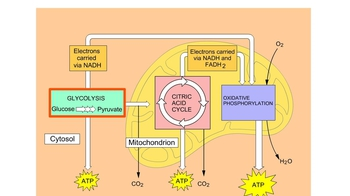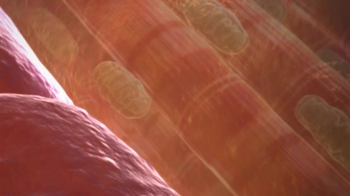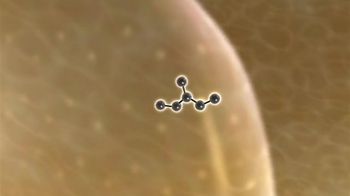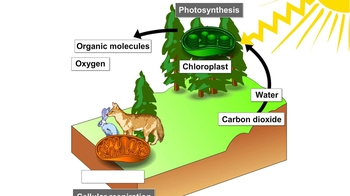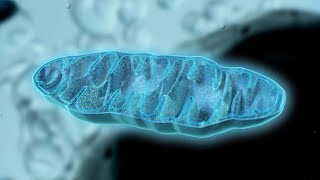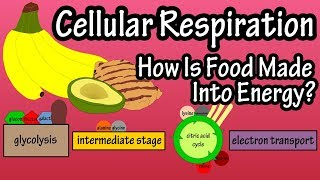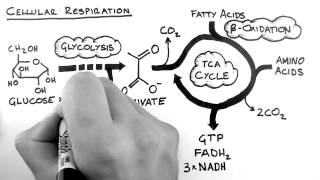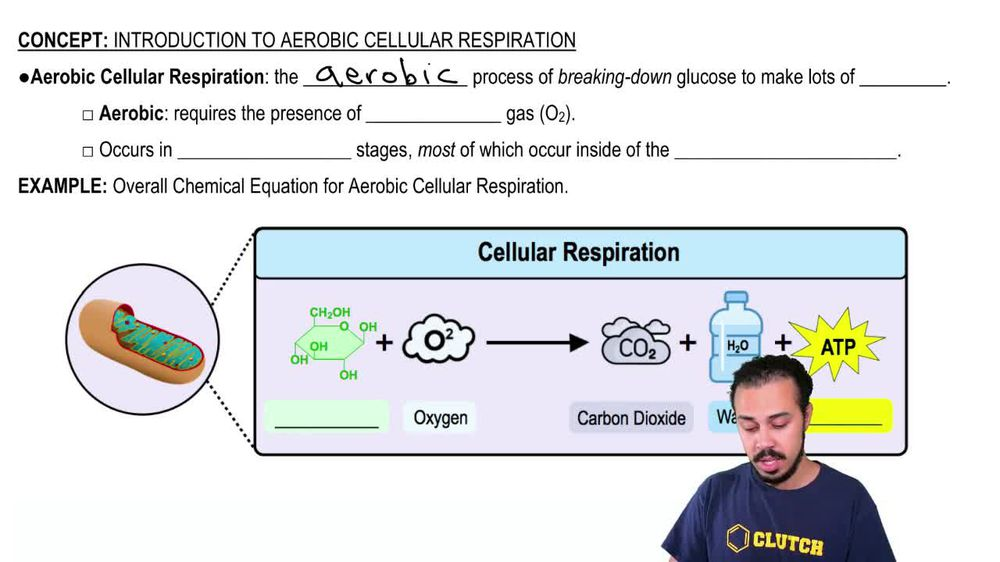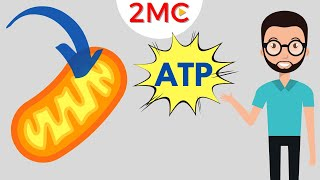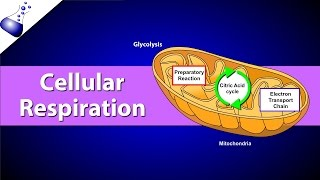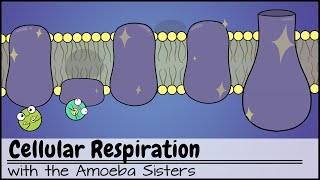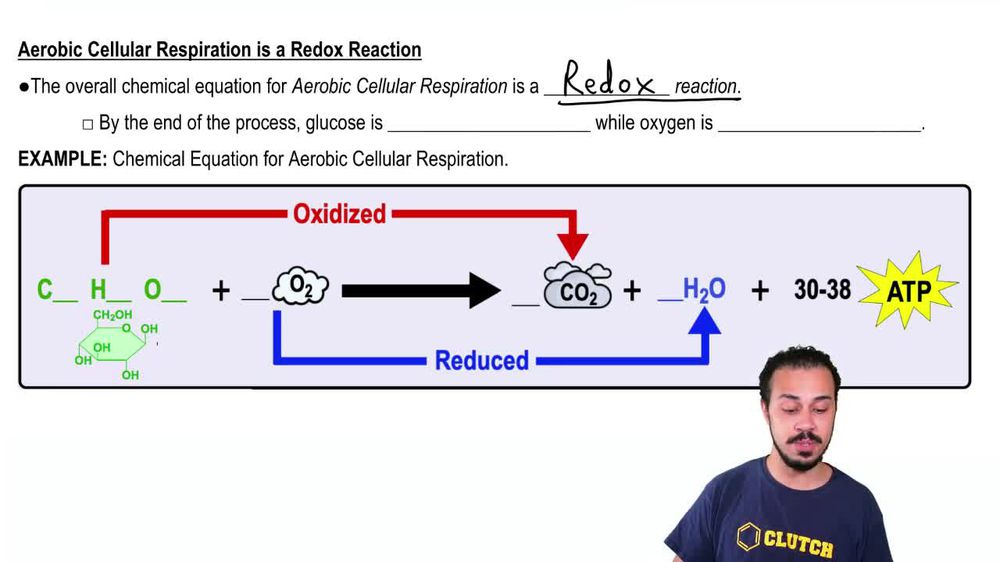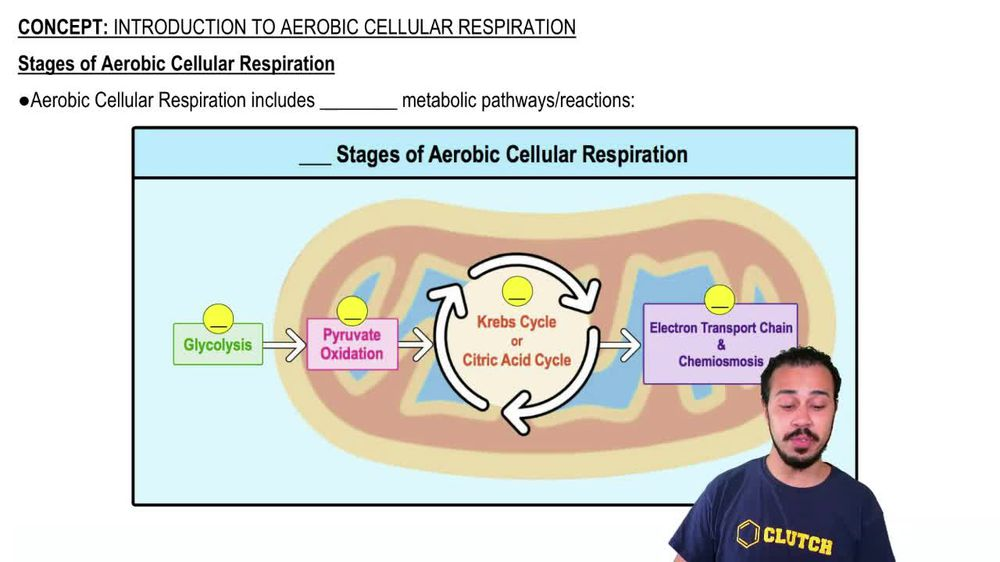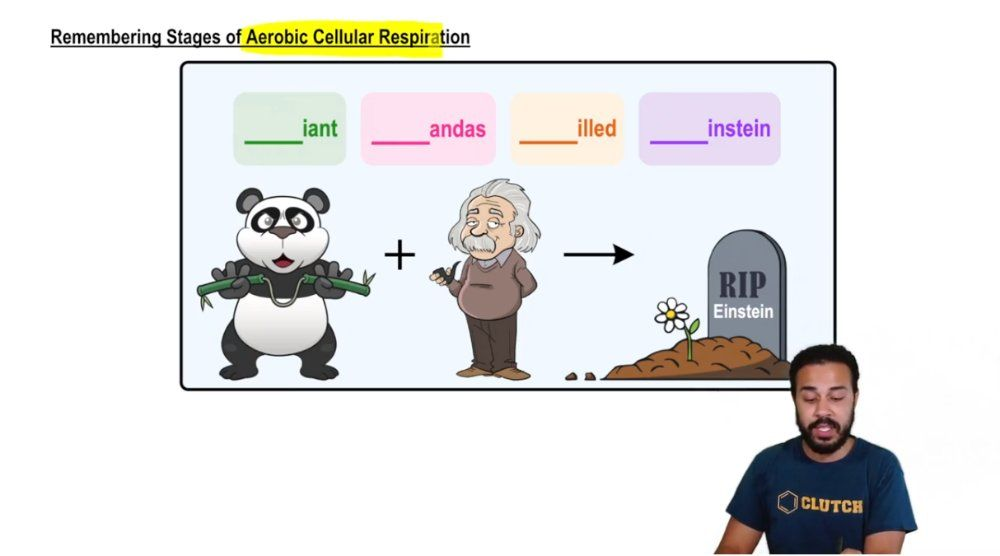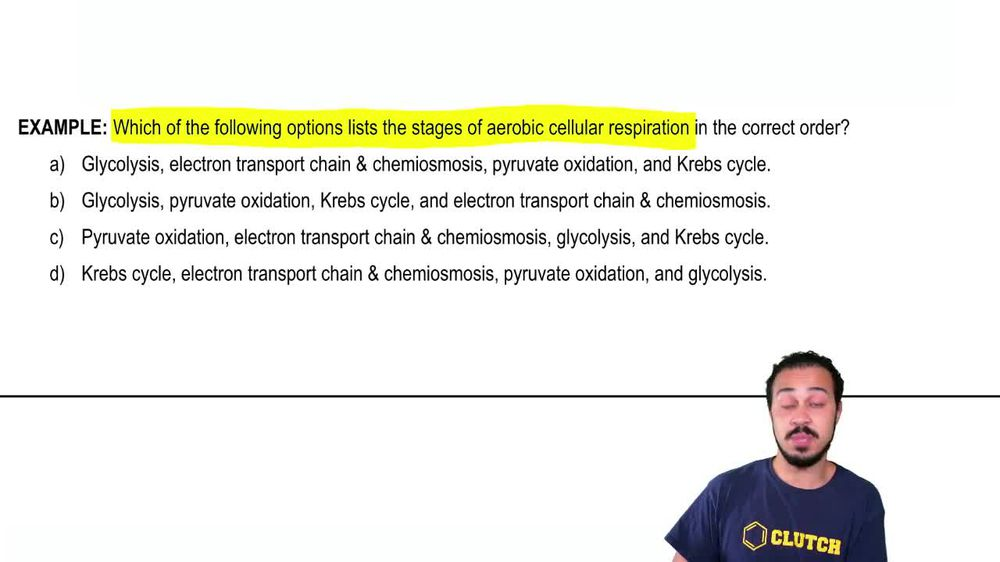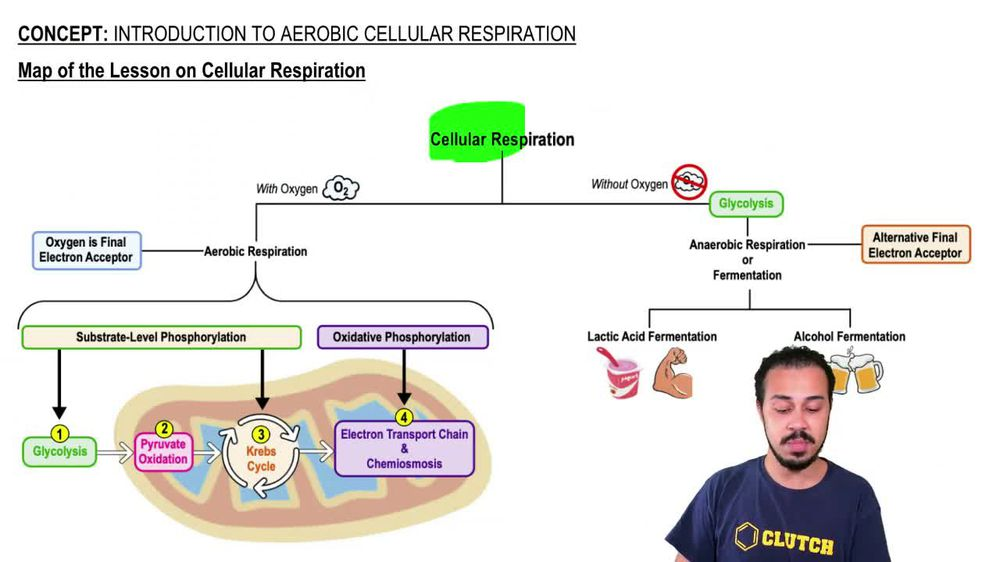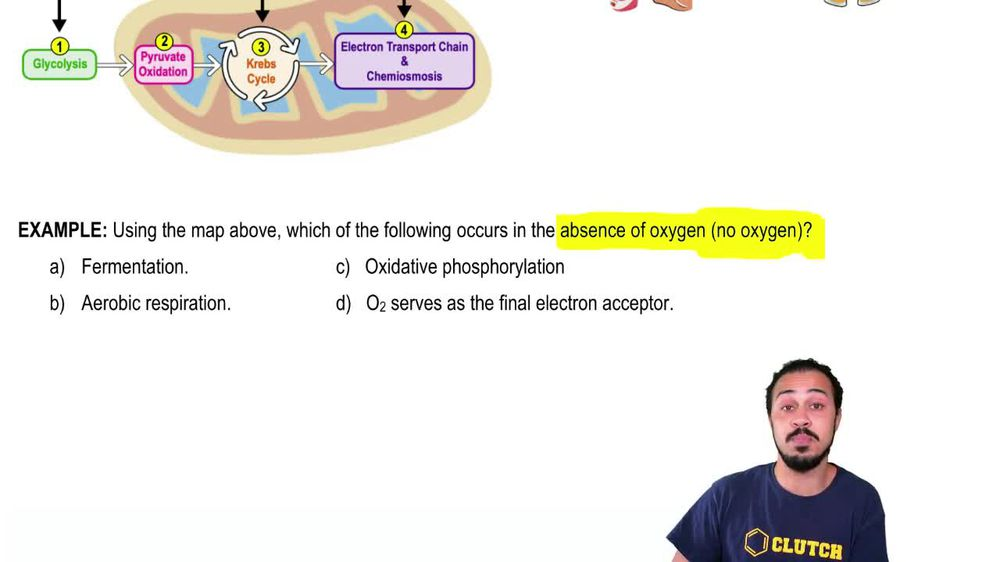Start typing, then use the up and down arrows to select an option from the list.
1. 8. Respiration2. Introduction to Cellular Respiration# Introduction to Cellular Respiration Example 2

by Jason Amores Sumpter
282 views
2
2
All right. So here we have an example problem that says using the map above which of the following occurs in the absence of oxygen or where no oxygen is present. And we've got these four potential answer options down below. And so when we take a look at our map of the lesson on cellular respiration from up above notice that this entire left hand branch over here is occurring in the presence of oxygen with oxygen being present, that's this entire left branch and noticed that the entire right branches occurring without oxygen, being present in the absence of oxygen. And so that is this entire right branch. And so notice. Once again, the problem is asking us which of the following occurs in the absence of oxygen where no oxygen is present. So it's specifically wants us to choose an option on the right hand side of our map. And so when we look at some of these options, such as aerobic respiration, oxidative foster relation and oxygen serving as the final Elektronik sector, notice that they all show up on the left hand side. Aerobic respiration is here. Oxidative foster relation is here, and oxygen serving as the final electron. Except er is here. And so those three things are all occurring with oxygen being present and the Onley one that occurs without oxygen. Being present is fermentation and so fermentation We could see over here on the right hand side. And there are two types that we're gonna talk about moving forward, lactic acid fermentation and alcohol fermentation. But for now, for this example problem. What we can see is that fermentation is going to be the correct answer here, the one that occurs in the absence of oxygen. And so we could go ahead and indicate that a Here is the correct answer to this example problem and that concludes this example. So I'll see you all in our next video.